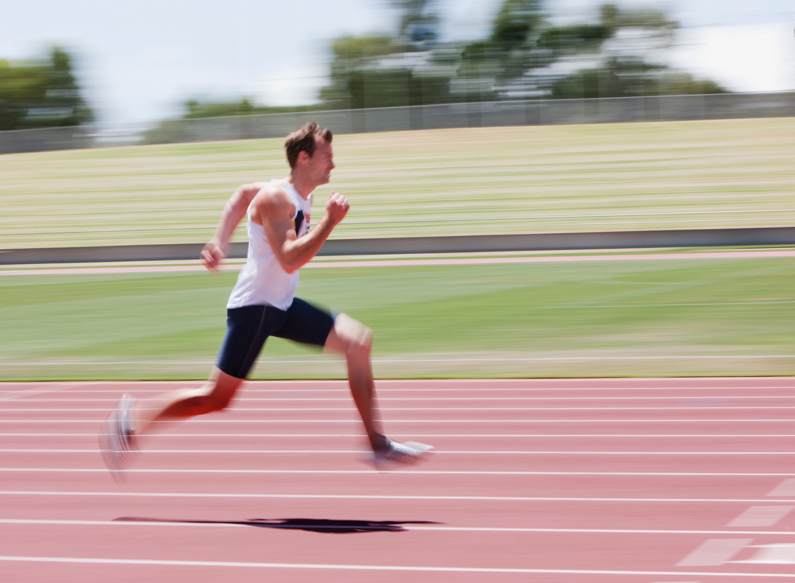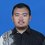# Help: Question from Indonesian OSN Guru Matematika SD

I need help regarding this question this question is from 2019 Indonesian National Science Olympiade for Mathematics Teacher. The question is ...Johnny and Diego are jogging on a circular track. Both start running at the same point. Johnny run with the speed of 50 km/h while Diego run with the speed of 80 km/h. after completing a lap, DIego rests for 30 minutes. After that he run again, and he meets Johnny. How long is the circumference of the track?Note by Ilham Syamsuddin
2 years, 1 month ago

This discussion board is a place to discuss our Daily Challenges and the math and science related to those challenges. Explanations are more than just a solution — they should explain the steps and thinking strategies that you used to obtain the solution. Comments should further the discussion of math and science.

When posting on Brilliant:

• Use the emojis to react to an explanation, whether you're congratulating a job well done , or just really confused .
• Ask specific questions about the challenge or the steps in somebody's explanation. Well-posed questions can add a lot to the discussion, but posting "I don't understand!" doesn't help anyone.
• Try to contribute something new to the discussion, whether it is an extension, generalization or other idea related to the challenge.

MarkdownAppears as
*italics* or _italics_ italics
**bold** or __bold__ bold
- bulleted- list
• bulleted
• list
1. numbered2. list
1. numbered
2. list
Note: you must add a full line of space before and after lists for them to show up correctly
paragraph 1paragraph 2

paragraph 1

paragraph 2

[example link](https://brilliant.org)example link
> This is a quote
This is a quote
    # I indented these lines
# 4 spaces, and now they show
# up as a code block.

print "hello world"
# I indented these lines
# 4 spaces, and now they show
# up as a code block.

print "hello world"
MathAppears as
Remember to wrap math in $$ ... $$ or $ ... $ to ensure proper formatting.
2 \times 3 $2 \times 3$
2^{34} $2^{34}$
a_{i-1} $a_{i-1}$
\frac{2}{3} $\frac{2}{3}$
\sqrt{2} $\sqrt{2}$
\sum_{i=1}^3 $\sum_{i=1}^3$
\sin \theta $\sin \theta$
\boxed{123} $\boxed{123}$

Sort by:

Thank you.

- 2 years, 1 month ago

- 2 years, 1 month ago

I find the wording "After that he runs again, and he meets Johnny" confusing, but if it just means that Johnny finishes 30 minutes later than Diego then I get an answer of 200/3 km as well. This is an unusually long track, (10.61 km radius), and the speeds of the two runners is beyond what humans are capable of (without superpowers).

- 2 years, 1 month ago

Yes. That's unimaginable.

- 2 years, 1 month ago

I got the answer as $\dfrac{200}{3} ~m$. Here is my solution :

Let the circumference of the track be $x$. Also, lets assume that Diego takes time $t$ (in hours) to completer one lap. It is given that he takes $0.5$ hours rest and then meets Johny. From this we can conclude that Johny takes $t + 0.5$ hours to complete the lap. We know their speeds so put them in the condition $x = v \cdot t$ where, v is their respective speeds.

$x = 80 \cdot t \\ x = 50 \cdot (t + 0.5)$ On solving we get $t = \dfrac56~hr= 50~min$ and $\boxed{x = \dfrac{200}{3}~km}$.

(But I can't imagine a track which has circumference almost equal to 67 Km)

- 2 years, 1 month ago